# ICSE Solutions for Selina Concise Chapter 10 Isosceles Triangles Class 9 Maths

### Exercise 10(A)

1. In the figure alongside,

AB = AC

∠A = 48o and

ACD = 18o.

Show that BC = CD.

But,
∠ACB = ∠ABC [AB = AC]
⇒ 2∠ABC = 180° - 48°
⇒ 2∠ABC = 132°
⇒ ∠ACB = 66° = ∠ACB ...(i)
⇒ ∠ACB = 66°
⇒ ∠ACD + ∠DCB = 66°
⇒ 18° +∠DCB = 66°
⇒ ∠DCB = 48° ...(ii)
Now In  △DCB,
∠DCB = 66° [From (i), Since ∠ABC = ∠DBC]
⇒ ∠DCB = 48° [From (ii)]
⇒ ∠BDC = 180° - 48° - 66°
⇒ ∠BDC = 66°
Since, ∠BDC =∠DBC
∴ BC= CD
Equal angles have equal sides opposite to them.

2. Calculate:

(ii) ∠ABC

(iii) ∠BAC

Proof:
(i) ∠ACD + ∠ACE = 180° [DCE is a st. line]
⇒ ∠ACD = 180° - 130°
⇒ ∠ACD = 50°
Now,
⇒∠ACD = ∠DAC = 50° ...(i) [Since angles opposite to equal sides are equal]

∠ACD = ∠DAC = 50°
∠ACD + ∠DAC + ∠ADC = 180°
50° + 50° + ∠ADC = 180°

(ii) ∠ADC = ∠ABD + ∠DAB  [Exterior angle is equal to sum of opp. interior angles]
∴ ∠DAB = ∠ABD
⇒ 80° = ∠ABD + ∠ABD
⇒ 2∠BD  = 80°
⇒ ∠ABD  = 40° = ∠DAB ...(ii)

(iii) ∠BAC = ∠DAB + ∠DAC
Substituting the values from (i) and  (ii)
∠BAC = 40° + 50°
⇒∠BAC = 90°

3. In the following figure, AB = AC; BC = CD and DE is parallel to BC. Calculate:

(i) ∠CDE

(ii) ∠ DCE

∠BAC + ∠FAB = 180°  [FAC is a straight line]
⇒ ∠BAC = 180° - 128°
⇒ ∠BAC = 52°
In △ABC,
∠A = 52°
∠B = ∠C  [Given AB = AC and angles opposite to equal sides are equal]
∠A +∠B +∠C = 180°
⇒∠A +∠B +∠B = 180°
⇒ 52° + 2∠B = 180°
⇒ 2∠B = 128°
⇒ ∠B = 64° = ∠C  ...(i)
∠B = ∠ADE  [Given DE∥ BC]

(i) Now,
⇒ 64° + ∠CDE + 64° = 180°
⇒ ∠CDE = 180° - 128°
⇒ ∠CDE = 52°

(ii) Given DE ∥ BC and DC is the transversal.
⇒∠CDE = ∠DCB = 52° ...(ii)
Also, ∠ECB = 64° ...[From  (i)]
But,
∠ECB = ∠DCE + ∠DCB
⇒ 64° = ∠DCE + 52 °
⇒ ∠DCE = 64° - 52°
⇒ ∠DCE = 12°

4. Calculate x in the given figure:

(i)

(i) Let the triangle be ABC and the altitude be AD.

Now,
∠CDA = ∠DBA + ∠DAB  [Exterior angle is equal to the sum of opp. interior angles]
∴ ∠CDA = 37° + 37°
⇒ ∠CDA = 74°
∠CDA = ∠CAD = 74°  [Given CD = AC and angles opposite to equal sides are equal]
Now,
∠CAD + ∠CDA + ∠ACD = 180°
⇒ 74° + 74° + x = 180°
⇒ x = 180° - 148°
⇒ x = 32°

(ii) Let triangle be ABC and altitude be AD.
Now,
∠CDA = ∠DBA + ∠DAB  [Exterior angle is equal to the sum of opp. interior angles]
⇒ ∠CDA = 50° + 50°
⇒ CDA = 100°
∠DAC = ∠DCA = x  [Given AD = DC and angles opposite to equal sides are equal]
∴ ∠DAC  + ∠DAC + ∠ADC = 180°
⇒ x+x+100° = 180°
⇒ 2x = 80°
⇒ x = 40°

5. In the figure, given below, AB = AC. Prove that: BOC = ACD. Answer
Since ∠B = ∠C  [ AB = AC]
⇒ (1/2)B = (1/2)C
⇒ x = y
Now,
∠ACD = 2x + ∠BAC  [Exterior angle is equal to sum of opp. interior angles]
⇒ ∠ACD = 2x + 180° - 2x -2y  [From (i)]
⇒ ∠ACD = 180° - 2y ...(ii)
In △OBC,
∠BOC = 180° - x - y
⇒ ∠BOC = 180° - y- y [Already proved]
⇒ ∠BOC = 180° - 2y ...(iii)
From (i) and (ii)
∠BOC = ∠ACD

6. In the figure given below, LM = LN; angle PLN = 110o. Calculate:

(i) ∠LMN

(ii) ∠MLN

Given: ∠PLN = 110°
(i) We know that the sum of the measure of all the angles of a quadrilateral is 360°.

∠QPL + ∠PLN +∠LNQ +∠NQP = 360°
⇒ 90° + 110° + ∠LNQ + 90° = 360°
⇒∠LNQ = 360° - 290°
⇒ ∠LNQ = 70°
⇒ ∠LNM = 70° ...(i)

In △LMN,
LM = LN  [Given]
∴ ∠LNM = ∠LMN  [angles opp. to equal sides are equal]
⇒ ∠LMN = 70° ...(ii) [ From (i)]

(ii) In △LMN,
∠LMN + ∠ LMN + ∠MLN = 180°
But, ∠LNM = ∠LMN = 70°  [From (i) and (ii)]
∴ 70° + 70°+ ∠NLN = 180°
⇒ ∠MLN = 180° - 140°
⇒ ∠MLN = 40°

7.An isosceles triangle ABC has AC = BC. CD bisects AB at D and CAB = 55o.

Find: (i) ∠DCB (ii) ∠CBD.

In △ABC,
∠CBA + ∠CAB + ∠ACB = 180°
but, ∠CAB = ∠CBA = 55°
⇒ 55°+ 55° + ∠ACB = 180°
⇒ ∠ACB = 180° - 110°
⇒ ∠ACB = 70°
Now,
In △ACD and △BCD,
AC = BC  [Given]
CD = CD  [Common]
AD = BD  [Given: CD bisects AB]
∴ △ACD ≅ △BCD
⇒ ∠DCA = ∠DCB
⇒ ∠DCB = ∠ACB/2 = 70°/2
⇒ ∠DCB = 35°

Let us name the figure as following :In △ABC,
∴ ∠ADC = ∠ACD  [angles opp. to equal sides are equal]
Now,
∠ADC = ∠DAB + ∠DBA  [Exterior angle is equal to the sum of opp. interior angles]
But,
∠DAB = ∠DBA  [Given: BD = DA]
⇒ 2∠DBA = 42°
⇒ ∠DBA = 21°
For x :
x =  ∠CBA + ∠BCA  [Exterior angle is equal to the sum of opp. interior angles]
We know that,
∠CBA = 21°
∠BCA = 42°
∴ x = 21° +42°
⇒ x = 63°

9. In the triangle ABC, BD bisects angle B and is perpendicular to AC. If the lengths of the sides of the triangle are expressed in terms of x and y as shown, find the values of x and y. Answer
In △ABD and △DBC,

BD = BD  [Common]
∠BDA = ∠BDC  [each equal to 90°]
∠ABD = ∠DBC  [BD bisects ∠ABC]
∴  △ABD ≅ △DBC  [ASA criterion]
Therefore,
x +1 =  y+2
⇒ x = y+1  ...(i)
and AB = BC
3x +1 = 5y - 2
Substituting the value of x from (i)
3(y+ 1) + 1 = 5y -2
⇒ 3y + 3 + 1 = 5y- 2
⇒ 3y + 4 = 5y - 2
⇒ 2y = 6
⇒ y = 3
Putting y = 3 in  (i)
x = 3+1
∴ x = 4

10. In the given figure; AE || BD, AC || ED and AB = AC. Find ∠a, ∠b and ∠c.
Let P and Q be the points as shown below :
∠PDQ = 58°
∠PDQ = ∠EDC = 58°  [Vertically opp. angles]
∠EDC = ∠ACB = 58°  [Corresponding angles ∵ AC॥ ED]
In △ABC,
AB = AC  [Given]
∴ ∠ACB = ∠ABC = 58° [angles opp. to equal sides are equal]
Now,
∠ACB + ∠ABC + ∠BAC = 180°
⇒ 58° +58° + a = 180°
⇒ a = 180° - 116°
⇒ a = 64°
Since AE॥ BD and AC is the transversal
∠ABC = b  [Corresponding angles]
∴ b = 58°
Also since AE॥ BD and ED is the transversal
∠EDC = c  [Corresponding angles]
∴ c = 58°

11. In the following figure; AC = CD, AD = BD and ∠C = 58o.

Find angle CAB.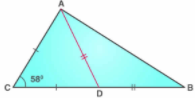In △ACD,
AC = CD  [Given]
∠ACD = 58° [Given]
∠ACD + ∠CDA + ∠CAD = 180°
⇒ 58° + 2∠CAD = 180°
⇒ ∠CAD = ∠CDA = 61° ...(i)
Now,
∠CDA = ∠DAB + ∠DBA  [Ext. angle is equal to sum of opp. int. angles]
But,
∠DAB = ∠DBA   [Given: AD = DB]
∴ ∠DAB + ∠DAB = ∠CDA
⇒ 2∠DAB = 61°
⇒ ∠DAB = 30.5° ...(ii)
In △ABC,
∴ ∠CAB = 61° + 30.5°
⇒ ∠AB = 91.5°

12. In the figure of q. no. 11 given above, if AC = AD = CD = BD; find angle ABC.

In △ACD,
AC = AD = CD  [Given]
Hence, ACD  is an equilateral triangle
∴ ∠ACD = ∠CDA = ∠CAD = 60°
∠CDA = ∠DAB + ∠ABD  [Ext. angle is equal to sum of opp. int. angles]
But,
∠DAB = ∠ABD  [Given: AD = DB]
∴ ∠ABD + ∠ABD = ∠CDA
⇒ 2∠ABD = 60°
⇒ ∠ABD = ∠ABC = 30°

13. In triangle ABC; AB = AC and ∠A : ∠B = 8 : 5; find angle A.Let ∠A = 8x and ∠B = 5x
Given: AB = AC
⇒∠B = ∠C = 5x  [Angles opp. to equal sides are equal]
Now,
∠A + ∠B +∠C = 180°
⇒ 8x +5x +5x = 180°
⇒ 18x = 180°
⇒ x = 10°
Given that:
∠A = 8x
⇒ ∠A = 8×10°
⇒ ∠A = 80°

14. In triangle ABC; ∠A = 60o, ∠C = 40o, and bisector of angle ABC meets side AC at point P. Show that BP = CP.
⇒ ∠B = 80°
Now,
BP is the bisector of  ∠ABC
∴ ∠PBC = ∠ABC/2
⇒ ∠PBC = 40°
In △PBC,
∠PBC = ∠PCB = 40°
∴ BP = CP  [Sides opp. to equal angles are equal]

15. In triangle ABC; angle ABC = 90o and P is a point on AC such that ∠PBC = ∠PCB. Show that: PA = PB.
∠ACB = x
⇒ ∠BAC = 180° - (90°+x)
⇒ ∠BAC = (90° - x) ...(i)
and
∠ABP = ∠ABC - ∠PBC
⇒ ∠ABP  = 90° - x ...(ii)
Therefore in the triangle ABP;
∠BAP = ∠ABP
Hence, PA = PB [sides opp. to equal angles are equal]

16. ABC is an equilateral triangle. Its side BC is produced upto point E such that C is mid-point of BE. Calculate the measure of angles ACE and AEC.
Answer △ABC is an equilateral triangle
⇒Side AB = Side AC
⇒ ∠ABC = ∠ACB [If two sides of a triangle are equal, then angles opposite to them are equal]
Similarly,
Side AC = Side BC
⇒ ∠CAB = ∠ABC [If two sides of a triangle are equal, then angles opposite to them are equal]
Hence,
∠ABC = ∠CAB = ∠ACB = y (say)
As the sum of all the angles of the triangle is 180°
∠ABC + ∠CAB + ∠ACB = 180°
⇒3y = 180°
⇒ y = 60°
∠ABC = ∠CAB = ∠ACB = 60°
Sum of two non-adjacent interior angles of a triangle is equal to the exterior angle.
⇒ ∠CAB + ∠CBA = ∠ACE
⇒ 60° + 60° = ∠ACE
⇒ ∠ACE = 120°
Now, triangle ACE is an isosceles triangle with AC = CF
⇒ ∠EAC = ∠AEC
Sum of all the angles of a triangle is 180°
∠EAC +∠AEC +∠ACE = 180°
⇒ 2∠AEC + 120° = 180°
⇒ 2∠AEC = 180° - 120°
⇒ ∠AEC = 30°

17. In triangle ABC, D is a point in AB such that AC = CD = DB. If ∠B = 28°, find the angle ACD.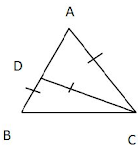△DBC is an isosceles triangle
As, Side CD  = Side DB
⇒ ∠DBC = ∠DCB  [If two sides of a triangle are equal, then angles opposite to them are equal]
And ∠B =∠DBC = ∠DCB = 28°
As the sum of all the angles of the triangle is 180°
∠DCB + ∠DBC + ∠BCD = 180°
⇒ 28° +28°+ ∠BCD = 180°
⇒ ∠BCD = 180° - 56°
⇒ ∠BCD = 124°
Sum of two non - adjacent interior angles of a triangle is equal to the exterior angle.
⇒ ∠DBC + ∠DCB = ∠DAC
⇒ 28° + 28° = 56°
⇒ ∠DAC = 56°
Now △ACD is an isosceles triangle  with AC = DC
⇒ ∠ADC = ∠DAC = 56°
Sum of all the angles of a triangle is 180°
∠ADC + ∠DAC + ∠DCA = 180°
⇒ 56° + 56° + ∠DCA = 180°
⇒ ∠DCA = 180° - 112°
⇒ ∠DCA = 64° = ∠ACD

18. In the given figure, AD = AB = AC, BD is parallel to CA and angle ACB = 65°. Find angle DAC.
Hence,
∠ABC = 65°
Sum of all the angles of a triangle is 180°
∠ACB + ∠CAB + ∠ABC = 180°
⇒ 65° + 65° +∠CAB = 180°
⇒ ∠CAB = 180°  - 130°
⇒ ∠CAB = 50°
As BD is parallel to CA
∴ ∠CAB = ∠DBA (Since they are alternate angles.)
∠CAB = ∠DBA = 50°
We see that triangle ADB is an isosceles triangle with side AD = Side AB .
Sum of all the angles of a triangle is 180°
⇒ ∠ADB  +∠DAB  + ∠DBA = 180°
⇒ 50°+∠DAB  + 50° = 180°
⇒ ∠DAB = 180° -100° = 80°
⇒ ∠DAB = 80°
The angle DAC is sum of angle DAB and CAB .
∠DAC = ∠CAB + ∠DAB
⇒ ∠DAC = 50° + 80°
⇒ ∠DAC = 130°

19. Prove that a triangle ABC is isosceles, if:

(i) altitude AD bisects angles BAC, or

(ii) bisector of angle BAC is perpendicular to base BC.

(i) In △ABC, let the altitude AD bisects ∠BAC.
Then we have to prove that the △ABC is isosceles.

⇒AB = AC  (c.p.c.t)
Hence, △ABC is an isosceles.

(ii) In △ABC, the bisector of ∠BAC is perpendicular to the base BC. We have to prove that the △ABC is isosceles.⇒AB = AC  (c.p.c.t)
Hence, △ABC is an isosceles.

20. In the given figure; AB = BC and AD = EC.

Prove that: BD = BE.In △ABC,
AB = BC  (given)
⇒ ∠BCA = ∠BAC  (angles opposite to equal sides are equal)
⇒ ∠BCD = ∠BAE  ...(i)
⇒ AD + DE = EC + DE  (Adding DE on both sides)
⇒ AE = CE  ....(ii)
Now, in triangles ABE and CBD
AB = BC  (given)
∠BAE = ∠BCD  [From (i)]
AE = CD  [From (ii)]
⇒ △ABE ≅ △CBD
⇒ BE  = BD  (c.p.c.t)

### Exercise 10(B)

1. If the equal sides of an isosceles triangle are produced, prove that the exterior angles so formed are obtuse and equal.Construction: AB is produced to D and AC is produced to E so that exterior angles ∠DBC and ∠ECB is formed.
In △ABC,
AB = AC  [Given]
∴ ∠C = ∠B  ...(i) [ angles opp. to equal sides are equal]
Since angle B and angle C are acute they cannot be right angles or obtuse angles.
∠ABC + ∠DBC  = 180°  [ABD is a straight line]
⇒ ∠DBC  = 180° - ∠ABC
⇒ ∠DBC = 180° - ∠B  ...(ii)
Similarly,
∠ACB + ∠ECB = 180° [ABD is a straight line]
⇒ ∠ECB = 180° -  ∠ACB
⇒ ∠ECB  = 180° - ∠C ...(iii)
⇒∠ECB = 180° - ∠B  ...(iv) [from (i) and (iii)]
⇒∠DBC = ∠ECB  [from (ii) and (iv)]
Now,
∠DBC = 180° - ∠B
But ∠B  = Acute angle
∴ ∠DBC = 180° - Acute angle = obtuse angle
Similarly,
∠ECB = 180° - ∠C,
But ∠C = Acute angle
∴∠ECB = 180° - Acute angle = obtuse angle
Therefore, exterior angles formed are obtuse and equal.

2. In the given figure, AB = AC. Prove that:

(i) DP = DQ

(ii) AP = AQIn △ABC,
AB = AC [Given]
∴ ∠C = ∠B  ...(i) [angles opp. to equal sides are equal]
(i) In △BPD and △CQD,
∠BPD  = ∠CQD [Each = 90°]
∠B = ∠C  [proved]
BD = DC  [Given]
∴△BPD ≅ △CQD [AAS critericn]
∴ DP = DQ  [c.p.c.t]

(ii) We have already proved that △BPD ≅ △CQD
Therefore, BP = CQ [c.p.c.t]
Now,
AB = AC [Given]
⇒ AB - BP = AC - CQ
⇒ AP = AQ

(iii) In △APD and △AQD,
DP = DQ  [proved]
AP = AQ [Proved]
∴ △APD  ≅ △AQD [SSS]

3. In triangle ABC, AB = AC; BE ⟂ AC and CF ⟂ AB.  Prove that:

(i) BE = CF

(ii) AF = AE

AB = AC  [Given]
⇒ △AEB ≅ △AFC  [AAS]
∴BE = CF  [c.p.c.t]

(ii) Since △AEB ≅ △AFC
∠ABE = ∠AFC
∴AF = AE  [congruent angles of congruent triangles]

4. In isosceles triangle ABC, AB = AC. The side BA is produced to D such that BA = AD. Prove that: ∠BCD = 90o.Construction: Join CD.
In △ABC,
AB = AC [Given]
∴ ∠C = ∠B  ...(i) [angles opp. to equal sides are equal]
In △ACD,
∠B + ∠ADC = ∠C + ∠ACD
⇒ ∠B + ∠ADC = ∠BCD ...(iii)
In △BCD,
∠B + ∠ADC + ∠BCD = 180°
⇒ ∠BCD + ∠BCD = 180°  [From (iii)]
⇒ 2∠BCD  = 180°
⇒ ∠BCD = 90°

5. (i) In triangle ABC, AB = AC and = 36°. If the internal bisector of ∠C meets AB at point D, prove that AD = BC.

(ii) If the bisector of an angle of a triangle bisects the opposite side, prove that the triangle is isosceles.

△ABC is an isosceles triangle.
∠A = 36°
∠B = ∠C = (180°-36°)/2  = 72°
∠ACD = ∠BCD = 36°  [CD is the angle bisector of ∠C]
△ADC is an isosceles triangle since ∠DAC = ∠DCA = 36°

(ii) In △DCB,
∠CDB = 180° - (∠DCB + ∠DBC)
= 180° - (36° + 72°)
= 180° - 108°
= 72°
△DCB is an isosceles triangle since ∠CDB = ∠CBD = 72°
∴ DC = BC ...(ii)
From (i) and (ii), we get
Hence, proved

6 . Prove that the bisectors of the base angles of an isosceles triangle are equal. AnswerIn △ABC,
AB = AC  [Given]
∴ ∠C = ∠B  ...(i) [angles opp. to equal sides are equal]
⇒(1/2)∠C = (1/2)∠B
⇒ ∠BCF = ∠CBE ...(ii)
In △BCE and △CBF,
∠C = ∠B  [From (i)]
∠BCF = ∠CBE  [From (ii)]
BC = BC [Common]
∴ △BCE ≅ △CBF  [AAS]
⇒BE = CF [c.p.c.t]

7. In the given figure, AB = AC and ∠DBC = ∠ECB = 90o

Prove that:

(i) BD = CE

In △ABC,
AB = AC [Given]
∴ ∠ACB = ∠ABC [angles opp. to equal sides are equal]
⇒∠ABC = ∠ACB ...(i)
∠DBC  =∠ECB = 90° [Given]

⇒∠DBC = ∠ECB ...(ii)
Subtracting (i) from (ii)
∠DCB - ∠ABC = ∠ECB - ∠ACB
⇒∠DBA = ∠ECA  ...(iii)

8. ABC and DBC are two isosceles triangles on the same side of BC. Prove that:

(i) DA (or AD) produced bisects BC at right angle.

(ii) ∠BDA = ∠CDA.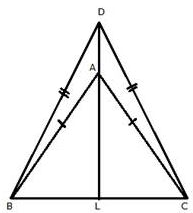DA is produced to meet BC in L.
In △ABC,
AB = AC  [Given]
∴∠ACB = ∠ABC ...(i) [angles opposite to equal sides are equal]
In △DBC,
DB = DC [Given]
∴∠DCB = ∠DBC ...(ii) [angles opposite to equal sides are equal]
subtracting (i) from (ii)
∠DCB - ∠ACB = ∠DBC - ∠ABC
⇒ ∠DCA = ∠DBA  ...(iii)
In △DBA and △DCA,
DB = DC  [Given]
∠DBA = ∠DCA  [From (iii)]
AB = AC  [Given]
∴△DBA ≅ △DCA  [SAS]
⇒ ∠BDA = ∠CDA  ....(iv) [c.p.c.t]
In △DBA
∠BAL = ∠DBA + ∠BDA  ...(v) [Ext. angle = sum of opp. int. angles]
From (iii), (iv) and (v)
∠BAL  = ∠DCA  + ∠CDA  ...(vi)
In △DCA,
∠CAL = ∠DCA + ∠CDA  ...(vii) [Ext. angle = sum of opp. int. angles]
From (vi) and (vii)
∠BAL = ∠CAL  ...(viii)
In △BAL and △CAL,
∠BAL = ∠CAL  [From  (viii)]
∠ABL = ∠ACL  [From (i)]
AB = AC  [Given]
∴△BAL ≅ △CAL  [ASA]
⇒ ∠ALB = ∠ALC  [c.p.c.t]
and BL = LC  ...(ix) [c.p.c.t]
Now,
∠ALB + ∠ALC = 180°
⇒ ∠ALB + ∠ALB = 180°
⇒ 2∠ALB = 180°
⇒ ∠ALB = 90°
∴ AL ⟂ BC
or DL ⟂ BC and BL = LC
∴ DA produced bisects BC at right angle.

9. The bisectors of the equal angles B and C of an isosceles triangle ABC meet at O. Prove that AO bisects angle A.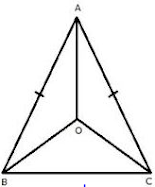In △ABC, we have AB = AC
⇒ ∠B = ∠C  [angles opposite to equal sides are equal]
⇒ (1/2)∠B  = (1/2)∠C
⇒ ∠OBC = ∠OCB  ...(i)
⇒ OB = OC ...(ii) [angles opposite to equal sides are equal]
Now,
In △ABO and △ACO,
AB = AC  [Given]
∠OBC = ∠OCB  [from (i)]
OB = OC  [From (ii)]
△ABO ≅ △ACO  [SAS criterion]
⇒∠BAO = ∠CAO  [c.p.c.t]
Therefore, AO bisects ∠BAC.

10. Prove that the medians corresponding to equal sides of an isosceles triangle are equal.
AB = AC [Given]
∴∠C = ∠B ...(i) [angles opp. to equal sides are equal]
⇒ (1/2)AB =  (1/2)AC
⇒ BF = CE ...(ii)
⇒ (1/2)AB = (1/2)AC
⇒ BF = CE ...(ii)
In △BCE and △CBF,
∠C = ∠B  [From (i)]
BF = CE [From (ii)]
BC = BC [Common]
∴△BCE ≅ △CBF   [SAS]
⇒ BE = CF [c.p.c.t]

11. Use the given figure to prove that, AB = AC.
AP = AQ  [Given]
∴∠APQ = ∠AQP  ...(i) [angles opposite to equal sides are equal]
In △ABP,
∠APQ = ∠BAP + ∠ABP  ...(ii) [Ext angle is equal to sum of opp. int. angles]
In △AQC,
∠AQP = ∠CAQ + ∠ACQ  ...(iii) [Ext angle is equal to sum of opp. int. angles]
From (i), (ii) and (iii)
∠BAP + ∠ABP = ∠CAQ + ∠ACQ
But, ∠BAP  = ∠CAQ  [Given]
⇒ ∠CAQ + ∠ABP = ∠CAQ + ∠ACQ
⇒ ∠ABP  = ∠CAQ + ∠ACQ - ∠CAQ
⇒ ∠ABP = ∠ACQ
⇒ ∠B =∠C ...(iv)
In △ABC,
∠B = ∠C
⇒ AB = AC  [Sides opposite to equal angles are equal]

12. In the given figure; AE bisects exterior angle CAD and AE is parallel to BC.

Prove that: AB = AC.Since AE ∥ BC and DAB is the transversal
∴∠DAE = ∠ABC  = ∠B [Corresponding angles ]
Since AE॥ BC and AC is the transversal
∠CAE  = ∠ACB = ∠C [Alternate Angles]
∴∠DAE = ∠CAE
⇒∠B = ∠C
⇒AB = AC [sides opposite to equal angles are equal]

13. In an equilateral triangle ABC; points P, Q and R are taken on the sides AB, BC and CA respectively such that AP = BQ = CR. Prove that triangle PQR is equilateral.
Answer AB = BC = CA  ...(i)  [Given]
AP = BQ  = CR  ...(ii) [ Given]
Subtracting (ii) from (i)
AB - AP = BC - BQ = CA = CR
BP = CQ = AR  ...(iii)
∴ ∠A = ∠B = ∠C ...(iv) [angles opp. to equal sides are equal]
In △BPQ and △CQR,
BP = CQ  [From (iii)]
∠B = ∠C  [From (iv)]
BQ = CR [Given]
∴ △BPQ ≅△CQR  [SAS criterion]
⇒ PQ = QR  ...(v)
In △CQR and △APR,
CQ = AR  [From (iii)]
∠C = ∠A  [From (iv)]
CR = AP  [Given]
∴ △CQR ≅ △APR  [ SAS criterion]
⇒ QR = PR ...(vi)
From (v) and (vi)
PQ = QR = PR
Therefore, PQR is an equilateral triangle.

14. In triangle ABC, altitudes BE and CF are equal. Prove that the triangle is isosceles.
BE = CF  [Given]
∴△ABE ≅  △ACF [AAS criteron]
⇒ AB = AC
Therefore, ABC is an isosceles triangle.

15. Through any point in the bisector of angle, a straight line is drawn parallel to either arm of the angle. Prove that the triangle so formed is isosceles.AL is bisector of angle A. Let D is any point on AL. From D, a straight line DE is drawn parallel to AC.
DE ॥ AC [Given]
∴∠ADE = ∠DAC ...(i) [Alternate angles]
∠DAC = ∠DAE  ...(ii) [AL is bisector of ∠A]
From (i) and (ii)
∴AE = ED    [sides opposite to equal angles are equal]
Therefore, AED is an isossceles triangle.

16. In triangle ABC; AB = AC. P, Q and R are mid-points of sides AB, AC and BC respectively. Prove that:

(i) PR = QR

(ii) BQ = CP

AB = AC
⇒ (1/2)AB = (1/2)AC
⇒ AP = AQ  ...(i) [since P and Q are mid - points]
In △BCA,
PR = (1/2)AC  [PR is line joining the mid - points of AB and BC]
⇒PR = AQ ...(ii)
In △CAB,
QR = (1/2)AB  [QR is line joining the mid - points of AC and BC]
⇒ QR = AP  ...(iii)
From (i), (ii) and (iii)
PR = QR

(ii)AB = AC
⇒ ∠B = ∠C
Also,
(1/2)AB = (1/2)AC
⇒ BP = CQ  [P and Q are mid - points of AB and AC]
In △BPC and △CQB,
BP = CQ
∠B = ∠C
BC = BC
Therefore, △BPC ≅ △CQB  [SAS]
BP = CP

17. From the following figure, prove that:

(i) ∠ACD = ∠CBE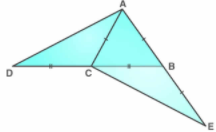(i) In △ACB,
AC = AC [Given]
∴∠ABC = ∠ACB ...(i) [angles opposite to equal sides are equal]
∠ACD + ∠ACB = 180 ...(ii) [DCB is a straight line]
∠ABC + ∠CBE = 180 ...(iii) [ABE is a straight line]
Equating (ii) and (iii)
∠ACD + ∠ACB = ∠ABC + ∠CBE
⇒∠ACD + ∠ACB = ∠ACB + ∠CBE  [From (i)]
⇒∠ACD = ∠CBE

(ii) In △ACD and △CBE,
DC = CB [Given]
AC = BE  [Given]
∠ACD = ∠CBE [Proved Earlier]
△ACD ≅ △CBE  [SAS criterion]

18. Equal sides AB and AC of an isosceles triangle ABC are produced. The bisectors of the exterior angle so formed meet at D. Prove that AD bisects angle A.
Answer AB is produced to E and AC is produced to F. BD is bisector of angle CBE and CD is bisector of angle BCF, BD and CD meet at D.
In △ABC,
AB = AC [Given]
∴ ∠C = ∠B  [angles opposite to equal sides are equal]
∠CBE = 180° - ∠B [ABE is a straight line ]
⇒ ∠CBD  =  (180° - ∠B)/2  [BD is bisector of ∠CBE]
⇒ ∠CBD = 90° - ∠B/2 ...(i)
Similarly,
∠BCF = 180° - ∠C [ACF is a straight line]
⇒ ∠BCD = (180° - ∠C)/2  [CD is bisector of ∠BCF]
⇒ ∠BCD = 90°  - ∠C/2  ...(ii)
Now,
⇒ ∠CBD = 90° - ∠C/2  [∵∠B = ∠C]
⇒ ∠CBD = ∠BCD
In △BCD,
∠CBD = ∠BCD
∴ BD = CD
In △ABD and △ACD,
AB = AC  [Given]
BD = CD  [Proved]
∴ △ABD ≅ △ACD  [SSS criterion]

19. ABC is a triangle. The bisector of the angle BCA meets AB in X. A point Y lies on CX such that AX = AY.

Prove that ∠CAY = ∠ABC.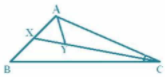In △ABC,
CX is the angle bisector of ∠C
⇒ ∠ACY = ∠BCX  ...(i)
In △ AXY,
AX = AY [Given]
∠AXY = ∠AYX  ...(ii) [angles opposite to equal sides are equal]
Now ∠XYC = ∠AXB = 180°  [straight line]
⇒ ∠AYX + ∠AYC = ∠AXY + ∠BXY
⇒ ∠AYC = ∠BXY  ...(iii) [From  (ii)]
In △AYC and △BXC
∠AYC + ∠ACY +∠CAY = ∠BXC + ∠BCX + ∠XBC = 180°
⇒ ∠CAY = ∠XBC [from (i) and (iii)]
⇒ ∠CAY = ∠ABC

20. In the following figure; IA and IB are bisectors of angles CAB and CBA respectively. CP is parallel to IA and CQ is parallel to IB.

Prove that:

PQ = The perimeter of the ∇ABC.

Answer Since IA ॥ CP and CA is a transversal
∴ ∠CAI = ∠PCA  [Alternate angles]
Also IA॥CP and AP is a transversal
∴ ∠IAB  = ∠APC [Corresponding angles]
But ∴ ∠CAI = ∠IAB [Given]
∴ ∠PCA = ∠APC
⇒ AC = AP
similarly,
BC = BQ
Now,
PQ = AP + AB + BQ
= AC + AB +BC
= Perimeter of △ABC

21. Sides AB and AC of a triangle ABC are equal. BC is produced through C upto a point D such that AC = CD. D and A are joined and produced upto point E. If angle BAE = 108o; find angle ADB.
But AB = AC
⇒ ∠3 = ∠2
⇒108° = ∠2 + ∠ADB ...(i)
Now,
In  ACD,
But AC = CD
Putting this value in (i)

22. The given figure shows an equilateral triangle ABC with each side 15 cm. Also, DE||BC, DF||AC and EG||AB.

If DE + DF + EG = 20 cm, find FG.

Answer ABC is an equilateral triangle.
Therefore, AB = BC= AC = 15 cm
∠A = ∠B = ∠C = 60°
In △ADE, DE ॥ BC [Given]
∠AED = 60° [∵ ∠ACB = 60°]
∠ADE = 60° [∵ ∠ABC = 60°]
∠DAE = 180° - (60° + 60°) = 60°
Similarly,
△BDF & △GEC are equilateral triangles.
= 60° [∵ ∠C = 60°]
Let AD = x, AE = x, DE = x [∵△ADE is an equilateral triangle]
Let BD = y , FD = y, FB = y [∵ △BDF is an equilateral triangle]
Let EC = z, GC = z, GE = z [∵ △GEC is an equilateral triangle]
Now, AD + DB = 15
⇒ x+ y = 15 ...(i)
AE + EC = 15 ⇒ X+Z = 15 ...(ii)
Given, DE + DF + EG =  20
⇒ X+Y+Z = 20
⇒ 15 +Z = 20 [from (i)]
⇒ Z = 5
From (ii), we get x = 10
∴ Y = 5
Also, BC = 15
BF + FG + GC = 15
⇒ Y + FG +Z = 15
⇒ 5 + FG + 5 = 15
⇒ FG = 5

23. If all the three altitudes of a triangle are equal, the triangle is equilateral. Prove it.
∴ △BEC ≅ △BFC [RHS]
⇒∠B = ∠C
Similarly,
∠A = ∠B
Hence, ∠A = ∠B = ∠C
⇒AB = BC = AC
Therefore, ABC is an equilateral triangle.

24. In a ABC, the internal bisector of angle A meets opposite side BC at point D. Through vertex C, line CE is drawn parallel to DA which meets BA produced at point E. Show that ACE is ∇ isosceles.
⇒∠1 = ∠4  ...(i) [corresponding angles]
∠2  = ∠3 ...(ii) [Alternate angles]
But ∠1 = ∠2 ...(iii) [AD is the bisector of ∠A]
From (i), (ii) and (iii)
∠3 =∠4
⇒ AC = AE
⇒ △ACE is an isosceles triangle.

25. In triangle ABC, bisector of angle BAC meets opposite side BC at point D. If BD = CD, prove that ABC is ∇isosceles.
In △ABD and △EDC,
BD = CD  [Given]
∠1 = ∠2  [vertically opposite angles]
∴△ABD ≅ △EDC [SAS]
⇒AB = CE  ...(i)
⇒AC = CE  ...(ii)
From (i) and (ii)
AB = AC
Hence, ABC is an isosceles triangle.

26. In △ABC, D is point on BC such that AB = AD = BD = DC. Show that:

∴ △ABD is an equilateral triangle.
= 180° - 60°
= 120°
∴∠1 = ∠2
But,
∠1 + ∠2 + ∠ADC = 180°
⇒ 2∠1 + 120° = 180°
⇒ 2∠1 = 60°
⇒ ∠1 = 30°
⇒ ∠C = 30°
∴ ∠ADC : ∠C = 120° : 30°
⇒ ∠ADC : ∠C = 4 : 1

27. Using the information given in each of the following figures, find the values of a and b.

[Given: CE = AC]

(i)
∠CAE = ∠AEC = (180°-68°)/2 = 56° [∵ CE = AC]
In ∠BEA,
a = 180° - 56° = 124°
In △ABE,
∠ABE = 180° - (a + ∠BAE)
= 180° - (124° + 14°)
= 180° - 138° = 42°

(ii)